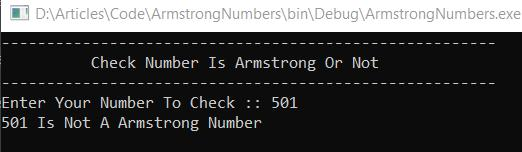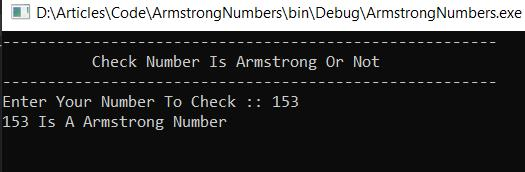Loading, please wait...# Check If Entered Number Is Armstrong Or Not In C# - Rejected - Plagarism found

In this article, we will create a program in c# to check whether the entered number is an Armstrong number or not.

## Introduction

In this article, we will create a program in c# to check whether the entered number is an Armstrong number or not.

## What is an Armstrong Number?

An Armstrong number is an n-digit number that is equal to the sum of the nth powers of its digits. A number that is the sum of its own digits each raised to the power of the number of digits. An Armstrong number of three digits is an integer where the sum of the cubes of its digits is equal to the number itself.

Let's try to understand why 371 is an Armstrong number.

371 = (3*3*3)+(7*7*7)+(1*1*1)

where:

(3*3*3)=27

(7*7*7)=343

(1*1*1)=1

So:

27+343+1=371

C# Code

``````using System;

namespace ArmstrongNumbers
{
class Program
{
static void Main(string[] args)
{
int number, rem, temp, sum = 0;
Console.WriteLine("-------------------------------------------------------");
Console.WriteLine("          Check Number Is Armstrong Or Not             ");
Console.WriteLine("-------------------------------------------------------");
Console.Write("Enter Your Number To Check :: ");

temp = number;
while (number > 0)
{
rem = number % 10;
sum =sum+(rem*rem*rem);
number= number/ 10;
}

if (temp == sum)
Console.WriteLine(temp + " Is A Armstrong Number");
else
Console.WriteLine(temp + " Is Not A Armstrong Number");
}
}
}  ``````
Code Explanation
1. First, we define four integer variable numbers, sum, rem, and temp then assign zero (0) to the sum.
2. Then get user input by Console.ReadLine() and pass this input to the number variable by converting it to an integer.
3. Now assign this user input number to our temp variable for future use.
4. Then we iterate while look until the number became zero(0).
5. In loop:
• First, we find the Remainder of the number by module its by 10.
• Then we get the power of this remainder by simply multiply it three times and add in our sum variable.
• Then assign the result of sum and power of remainder to the sum variable.
• Last, we divide the number by 10.
6. After a loop, check if the temp variable and sum variable are equal. If both are equal, then it's an Armstrong number, otherwise not.
7. Then we use the Console.ReadKey() method to read any key from the console. By entering this line of code, the program will wait and not exit immediately. The program will wait for the user to enter any key before finally exiting. If you don't include this statement in the code, the program will exit as soon as it is run.
Output